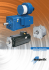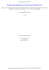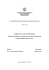# ch29_Magnetic_Induct..

## Transcription

ch29_Magnetic_Induct..
```Chapter 29
Magnetic Induction
Dr. Ray Kwok
SJSU
Static Fields (stationary charges, steady current)
 r r Qt 
∫ E ⋅ da = ε  Gauss’s Law
o
 r r

 E ⋅ d l = 0  Conservative
∫ r

r
 B ⋅ da = 0  No magnetic charge
∫

r
r
 B ⋅ d l = µ I  Ampere’s Law
o t
∫
linear, homogeneous, isotropic
Qt = Q f + Qb =
Qf
εr
≤ Qf
Orsted’s Discovery
R
4/21/1820
Danmark
V
I
compass
S
Hans Christian Ørsted
(1777–1851) Danmark
cgs unit for B
r r
∫ B ⋅ d l = µoIt
André-Marie Ampère
(1775–1836) France
SI unit for current
Ampere’s Law, 9/18/1820,
discovery on 9/11/1820 !!
With stationary magnet,
no current induced (1831)
(1791–1867) England
SI unit for capacitance
Joseph Henry
(1797–1878) USA
SI unit for inductance
Magnetic induction
r r
dΦ
Vemf ≡ ∫ E ⋅ d l = −
dt
r r
Φ = ∫ B ⋅ da
oppose the “change” of
magnetic flux
x
Φ = ∫ Bldx = Blx
0
B
l
R
I
x
u
dΦ
dx
= Bl
= Blu = −Vemf
dt
dt
Vemf Blu
I=
=
R
R
direction given by Lenz’s Law
Lenz’s Law (1834)
“Back emf” to oppose the “change” of magnetic flux
B
R
I
u
x
Heinrich Friedrich Emil Lenz
(1804-1865) Italy
Example – moving magnet
Example - jumping ring
FB
FB B
I
Al ring
I
R
V
S
e.g. magnet in a copper tube
S
N
Bind
Eddy current - disc brake
Lenz’s Law
metal plate
r
r r
FB = IL × B
To reduce eddy current
transformer
Example – metal detector
Example – guitar pickup
Question
Find the direction of the current in the resistor R shown in Figure at
each the following steps: (a) at the instant the switch is closed, (b)
after the switch has been closed for several minutes, (c) when the
variable resistance r increases, (d) when the circuit containing R
moving to the right, away from the other circuit, and (e) at the instant
the switch is opened.
r
S
R
V
Right, 0, Left, Left, Left
Moving Conductor
FB
+
r rr
FB=qu×B
B
saturate when
u
FB
−
induced
emf
B
R
r r
FE + FB = 0 separate the charges
r
r r
qE + qu × B = 0 induced E
r
r r
E = −u × B similar to Hall Effect
V
= uB
l
V = uBl
induced current
+
I = |V/R| = B u/R
l
I
u
-
direction agrees w/ Lenz’s Law
emf is due to the FB,
no E field build up.
Example – rotating loop
θ = ωt
uniform B
d r r
Vemf = − ∫ B ⋅ da
dt
d
= − ( BA cos ωt ) = ωBA sin ωt
dt
AC generator
dΦ
Vemf = − N
dt
= NωBA sin ωt
DC generator
Electromotor
Project 1: Make one in two weeks.
To turn faster, should we
1.
2.
3.
4.
use thicker wire?
use more turns?
make bigger loop?
use stronger magnet?
dΦ
= NωBA sin ωt
dt
NωBA
| I |=
R
r
r r
FB = I l × B
r r
r r r
τ = r × FB = m × B torque
r
magnetic moment
m = NIAn̂
r r
I = moment of inertia
τ = Iα
Vemf = − N
l
resistivity
A'
m = ρV = ρA' l mass density
R =ρ
1. use thicker wire?
same.
e.g. half R, double mass, same α
2. use more turns?
same.
double N, double R, same I,
double mm, but double inertia, same α.
3. use bigger loop? (same N)
same.
bigger A, more Φ
somewhat higher R, but still more I ~A/R~ r
mm~A2/R~r3, inertia~r3, same α
4. use stronger magnet?
YES
more I, m, τ, α
Another important application
– telephony / loudspeaker
(?? before him)
Alexander Graham Bell
(1847 –1922) UK
(practical improvement)
Thomas Alva Edison
(1847 –1931) USA
Ideal Transformer (AC)
dΦ
dt
dΦ
V2 = − N 2
dt
V1 N1
=
Voltage transformer
V2 N 2
Φ is confined in the core (µ = ∞) V1 = − N1
P1 = P2
RL
V1I1 = V2 I 2
V1 I 2 N1
= =
V2 I1 N 2
2
2
V1 V2  N1   N1 

 = 
 R L
R in =
=
I1
I2  N 2   N 2 
Impedance transformer
Self-Inductance
back emf causes I lags V
NΦ
L≡
I
LI = NΦ
dΦ
dI
V = −N
= −L
dt
dt
Ideal Solenoid
ideal:
• large N tightly wound
• no end effect
• uniform internal B
• zero external B in the vicinity
Ampere’s Law:
µ o NI
= µ o nI
B=
l
r r
Φ = ∫ B ⋅ da =µ o nIA
NΦ Nµ o nIA
L=
=
= µ o n 2lA
I
I
LC Resonator (Lenz’s Law, later resonator…)
Transformer -
Primary / Secondary coils
same cross-section?
V1 V2
∂Φ
=
=−
N1 N 2
∂t
N 2 N1 M12
=
=
I1
I 2 Φ12
same equations
different cross-section?
Electrodynamics
 r r Qt 
 ∫ E ⋅ da = ε

o


dΦ 
 r r
∫ E ⋅ d l = −


dt 
 r r

 ∫ B ⋅ da = 0

 r r

 ∫ B ⋅ d l = µ o I t 
Gauss’s Law
No magnetic charge
Ampere’s Law
Exercise
Rectangular loop of 20 turns is placed at 1 m
away from a long current-carrying wire as
shown.
I2
N = 20
I1(t) = 2 cos(60t) (A)
Total resistance of the wire in the loop is 4 Ω.
5m
Find I2.
I1(t)
3m
1m
```

### Formula Sheet for LSU Physics 2113, Third Exam, Fall ’14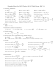### Dipartimento di Lingue e Letterature Straniere Dipartimento di Scienze Giuridiche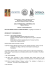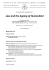### EU Energy Law &amp; Policy Workshop Decentralised Energy Law Enforcement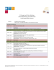### tax litigation - bkh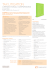### le fonti del diritto internazionale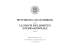### President`s Message/ Messaggio del Presidente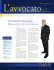### imp brochure lex ok.indd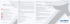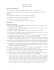### sensori magnetici serie smc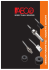### Z! - Argot ou La Maison Mobile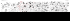### food design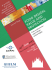### The Perfect Gift! CLICK TO WIN! Check out the coolest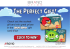### CineFiles Document #41306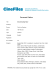### Downtown Boone Map 35x48-FINAL 02-24-15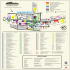### LSN Research into Top 100 UK Law Firm Directors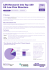### Applications of Magnetism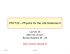### Lecture 13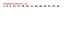### international conference on the legal status of roma and sinti in italy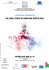### CATALOGO GENERALE ombre:Layout 1